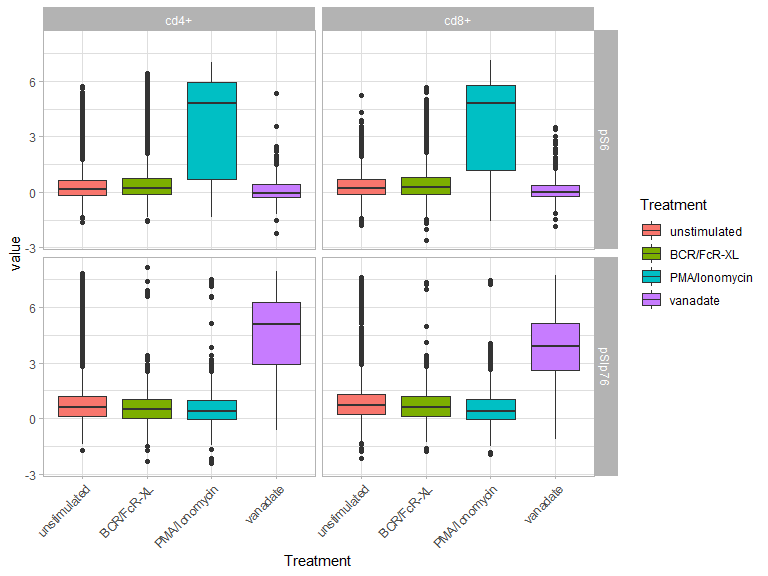# Abstract

The Radviz package implements the concept of dimensional anchors to visualize multivariate datasets in a 2D projection, as originally described in Hoffman et al 1999. Additional work for ordering of dimensional anchor is taken from Di Caro et al 2012.

# Introduction

So called big data has focused our attention on datasets that comprise a large number of items (or things). As a consequence the fact that we are measuring (or recording) more and more parameters (or stuff) is often overlooked, even though this large number of things is enabling us to explore the relationships between the different stuff with unprecedented efficacy.

Yet, this increase in the stuff we measure comes with a so-called Curse of Dimensionality: as our brains are unable to efficiently deal with more than 3 dimensions, we need new tools to visually explore larger (more than 3 dimensions) sets.

Several such tools exist that rely on projection into lower number of dimensions, such as PCA. Although those tools represent an efficient solution, they do not allow for direct interpretation of the position of the points in space.

Radviz provides an elegant solution to this problem, by projecting a N-dimensional data set into a simple 2D space where the influence of each dimension can be interpreted as a balance between the influence of all dimensions.

In Radviz, each dimension in the dataset is represented by a dimensional anchor, and each dimensional anchor is distributed evenly on a unit circle. Each line in the data set corresponds to a point in the projection, that is linked to every dimensional anchor by a spring. Each spring’s stiffness corresponds to the value for that particular thing in that particular dimension. The position of the point is defined as the point in the 2D space where the spring’s tension is minimum.

## Installation

The package can be installed using the following command:

install.packages('Radviz')

Once installed the package can be loaded using the standard library command.

library(Radviz)

library(ggplot2)
library(dplyr)
library(tidyr)

# Visualizing High Dimensional Data: the bodenmiller dataset

We will use data from the Bodenmiller et al 2012 publication. The bodenmiller package contains a subset of the data that can be used for testing purpose. The package can be installed by running the following command:

install.packages('bodenmiller')

Data has already been cleaned and transformed, and can be used as is.

library(bodenmiller)
data(refPhenoMat)
data(refFuncMat)
data(refAnnots)
ref.df <- data.frame(refAnnots,
refPhenoMat,
refFuncMat)

## Normalizing the data

Radviz requires that all dimensions have the same range. The simplest way to achieve that is to normalize every numerical column to its range, so that all values fall in the [0,1] interval. The do.L function performs this operation for us:

trans <- function(coln) do.L(coln,fun=function(x) quantile(x,c(0.005,0.995)))

The function is wrapped so that it can be applied directly within the do.radviz function. The extra argument fun is used to remove the top and bottom of each distribution which correspond to outliers:

hist(ref.df$CD3) abline(v=quantile(ref.df$CD3,c(0.005,0.995)),
col=2,lty=2)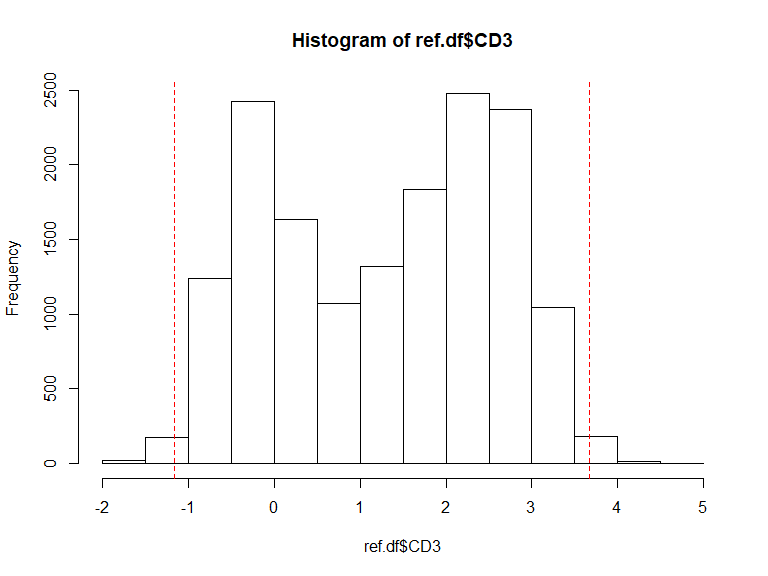## Defining the anchors

Let’s define a Spring object that contains the position of each dimension on the unit circle. The dimensions will be matched by column name to the data, so it is important to make sure that column names are syntactically valid, using the make.names function.

The make.S function will take a vector of column names and return a matrix of [x,y] positions. The order of the column names will dictate the position on the circle, starting at 12:00 and going clockwise. It is not required to use all available columns: it might be worth splitting the dimensions depending on what they represent (continuous versus categorical variables, phenotypic versus functional markers, etc.).

ct.S <- make.S(dimnames(refPhenoMat)[])

## Optimizing the position of anchors

The position of dimensional anchors on the circle is critical: the best projection (as judged by the separation of points in the display) is achieved when dimensional anchors that correspond to highly correlated dimensions in the data are placed closer on the unit circle.

Optimization of the position of dimensional anchors is discussed in Di Caro et al 2012, where the authors suggest 2 metrics that can be used in an optimization process. Both methods start with a similarity matrix where every value represents the similarity between any 2 dimensions. The first method assumes that dimensional anchors corresponding to similar dimensions should be close on the circle (radviz-independent); the second method uses a projection of the similarity matrix in Radviz and computes the distance of the dimensional anchors to the projected dimensions they represent (radviz-dependent) Di Caro et al 2012.

The Radviz-dependent and independent methods have been implemented in the Radviz package, as well as the recommended cosine similarity measure.

## compute the similarity matrix
ct.sim <- cosine(as.matrix(ref.df[,row.names(ct.S)]))
## the current Radviz-independent measure of projection efficiency
in.da(ct.S,ct.sim)
##  -8.771893
## the current Radviz-independent measure of projection efficiency
rv.da(ct.S,ct.sim)
##  6.104379

The Radviz-independent score should be maximal when the dimensional anchor positions are optimal. The Radviz-dependent score should be minimal when the dimensional anchor positions are optimal.

The optimization procedure works as follows:

optim.ct <- do.optimRadviz(ct.S,ct.sim,iter=100,n=1000)
## Selected optimization function: in.da
## Starting performance: -8.771893
## 0 Current performance: -10.78042
## 1 Current performance: -10.8634
## 2 Current performance: -10.8634
## 3 Current performance: -10.94059
## 4 Current performance: -10.94059
## 5 Current performance: -10.94059
## 6 Current performance: -10.94059
## 7 Current performance: -10.94059
## 8 Current performance: -10.94059
## Execution stopped after 8 iterations: no better solution found in the last 5 iterations
ct.S <- make.S(get.optim(optim.ct))

The original anchor position corresponds to the order of the columns in the matrix:

• CD20
• IgM
• CD4
• CD33
• HLA.DR
• CD14
• CD7
• CD3
• CD123

The optimized anchors are:

• CD123
• CD14
• CD4
• CD3
• CD7
• IgM
• CD20
• HLA.DR
• CD33

It is possible to rotate the anchors so that a particular dimension is at the top of the circle, using the function recenter:

ct.S <- recenter(ct.S,'CD3')

• CD3
• CD7
• IgM
• CD20
• HLA.DR
• CD33
• CD123
• CD14
• CD4

## Projection

The do.radviz function will then project values in the context of the Springs:

ct.rv <- do.radviz(ref.df,ct.S,trans=trans)

do.radviz will scale all values to [0,1] using do.L by default.

## Visualizing the results

Internally the Radviz projection is a ggplot object. Details about the data and corresponding projection are available through Radviz-specific versions of S3 generic functions:

summary(ct.rv)
## A Radviz object with 15792 objects and 9 dimensions
##                              Source       Cells        CD20         IgM
##           CD4     CD33     HLA.DR       CD14         CD7       CD3      CD123
## 1  0.24538905 1.845812 0.28467544  0.7867635  0.05080669 0.8882771 -0.1736830
## 2  0.18752959 2.276282 0.43561827  1.0316463 -0.54740331 0.8721281  0.4542218
## 3 -0.02593398 2.119974 0.75633795 -0.1576259  2.72629430 1.7181924 -0.0667001
## 4 -0.05774378 1.750508 0.73147988  0.1632028  2.78216921 0.2802657  0.3633580
## 5 -0.13402830 1.511281 0.69828572  0.2260039  3.38965411 0.2092649  0.6444306
## 6  2.34379280 1.819468 0.06367918 -0.3567477  2.09006275 1.5602333 -0.2513046
##          pStat1      pSlp76      pBtk    pPlcg2     pErk      pLat         pS6
## 1  1.5543929594  0.39820594 2.1455563 0.4076647 2.127705 0.7863917  0.45575696
## 2  2.2617867890  2.24430221 1.6472855 2.6780887 2.314259 1.7699250  0.90970326
## 3  0.9087872965  1.51443383 1.2106579 1.0207694 0.840385 1.8158869 -0.11516882
## 4 -0.0009201049  0.52799515 1.9187885 3.1896712 3.167694 0.5876629  1.22837250
## 5  0.8515650817 -0.00958695 0.9095552 0.6047778 2.036200 0.1807284 -0.09090411
## 6  1.7630741701  6.42808067 3.4870008 7.8620725 3.326642 2.9241200  0.47933446
##       pNFkB         pp38     pStat5     pAkt       pSHP2       pZap70
## 1 2.2061063 -0.192718118  1.3481375 1.591763  0.61411340  1.705732795
## 2 1.1788794  1.018837991  2.0934835 1.407424  0.21597453  1.403636251
## 3 1.4109075 -0.003533928 -0.1503696 2.771222 -0.07459359 -0.006048547
## 4 0.8870827  0.203404086  2.3198857 2.197841 -0.04034245  1.473085758
## 5 0.6636323  1.424795962  0.1999124 2.320152  0.66673986  1.418054027
## 6 1.8517821  3.045412563  3.5175258 2.638554  3.61195736  2.378537517
##       pStat3           rx           ry rvalid
## 1  0.3523257 -0.093928941 -0.114732650  FALSE
## 2 -0.1021022 -0.234579217 -0.170415001  FALSE
## 3  0.5538451  0.057077028  0.066544234  FALSE
## 4 -0.1495533 -0.025033351 -0.019598471  FALSE
## 5  0.7728979  0.006234689  0.006631637  FALSE
## 6  3.1662909 -0.104975983  0.219860655  FALSE
## Dimensional Anchors are CD3 CD7 IgM CD20 HLA.DR CD33 ...
head(ct.rv)
##                              Source       Cells        CD20         IgM
##           CD4     CD33     HLA.DR       CD14         CD7       CD3      CD123
## 1  0.24538905 1.845812 0.28467544  0.7867635  0.05080669 0.8882771 -0.1736830
## 2  0.18752959 2.276282 0.43561827  1.0316463 -0.54740331 0.8721281  0.4542218
## 3 -0.02593398 2.119974 0.75633795 -0.1576259  2.72629430 1.7181924 -0.0667001
## 4 -0.05774378 1.750508 0.73147988  0.1632028  2.78216921 0.2802657  0.3633580
## 5 -0.13402830 1.511281 0.69828572  0.2260039  3.38965411 0.2092649  0.6444306
## 6  2.34379280 1.819468 0.06367918 -0.3567477  2.09006275 1.5602333 -0.2513046
##          pStat1      pSlp76      pBtk    pPlcg2     pErk      pLat         pS6
## 1  1.5543929594  0.39820594 2.1455563 0.4076647 2.127705 0.7863917  0.45575696
## 2  2.2617867890  2.24430221 1.6472855 2.6780887 2.314259 1.7699250  0.90970326
## 3  0.9087872965  1.51443383 1.2106579 1.0207694 0.840385 1.8158869 -0.11516882
## 4 -0.0009201049  0.52799515 1.9187885 3.1896712 3.167694 0.5876629  1.22837250
## 5  0.8515650817 -0.00958695 0.9095552 0.6047778 2.036200 0.1807284 -0.09090411
## 6  1.7630741701  6.42808067 3.4870008 7.8620725 3.326642 2.9241200  0.47933446
##       pNFkB         pp38     pStat5     pAkt       pSHP2       pZap70
## 1 2.2061063 -0.192718118  1.3481375 1.591763  0.61411340  1.705732795
## 2 1.1788794  1.018837991  2.0934835 1.407424  0.21597453  1.403636251
## 3 1.4109075 -0.003533928 -0.1503696 2.771222 -0.07459359 -0.006048547
## 4 0.8870827  0.203404086  2.3198857 2.197841 -0.04034245  1.473085758
## 5 0.6636323  1.424795962  0.1999124 2.320152  0.66673986  1.418054027
## 6 1.8517821  3.045412563  3.5175258 2.638554  3.61195736  2.378537517
##       pStat3           rx           ry rvalid
## 1  0.3523257 -0.093928941 -0.114732650  FALSE
## 2 -0.1021022 -0.234579217 -0.170415001  FALSE
## 3  0.5538451  0.057077028  0.066544234  FALSE
## 4 -0.1495533 -0.025033351 -0.019598471  FALSE
## 5  0.7728979  0.006234689  0.006631637  FALSE
## 6  3.1662909 -0.104975983  0.219860655  FALSE
dim(ct.rv)
##  15792     9

The print.radviz S3 method shows the dimensional anchors:

ct.rv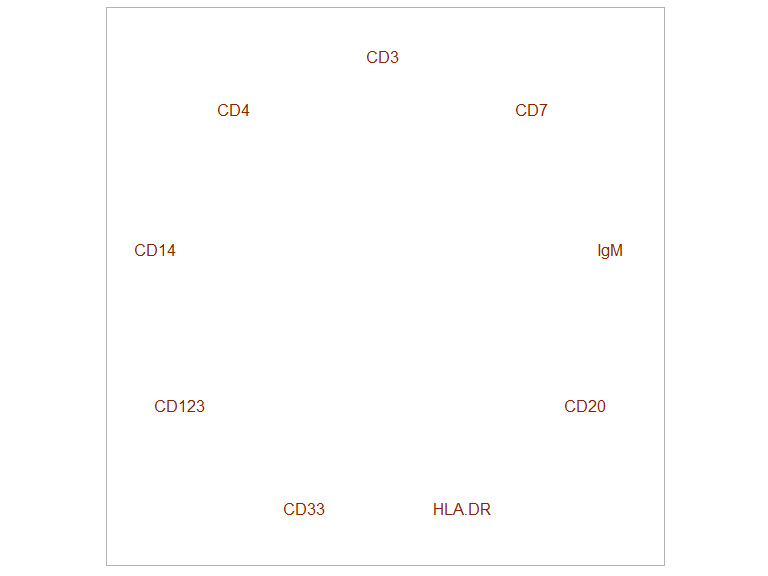To visualize the projected points one should use the plot.radviz method:

plot(ct.rv,anchors.only=FALSE)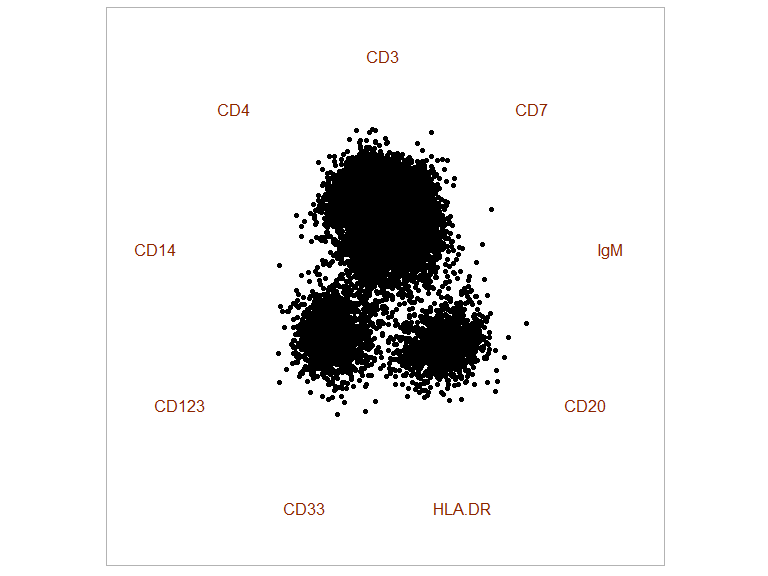By default the plot.radviz function will only show the anchors and return the ggplot object so that other layers can be added:

plot(ct.rv)+
geom_point()From there on all ggplot2 geoms and options are available:

plot(ct.rv)+
geom_point(data=. %>%
arrange(CD4),
aes(color=CD4))+
scale_color_gradient(low='grey80',high="dodgerblue4")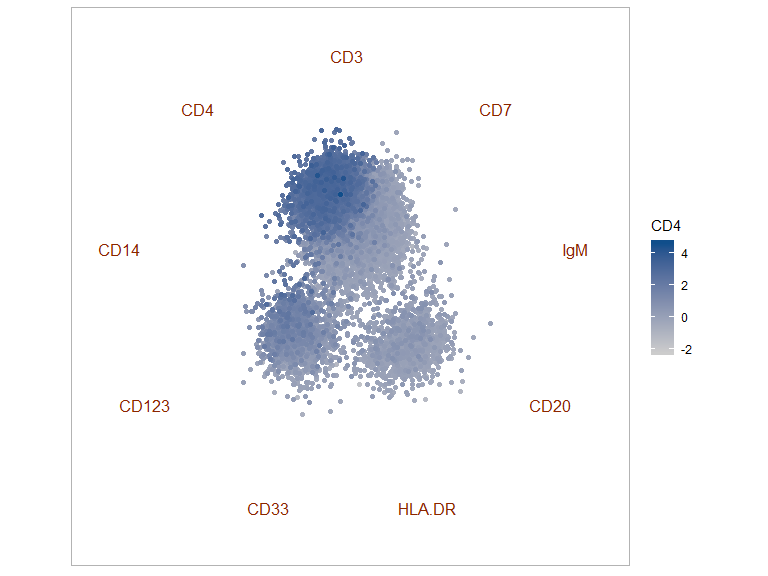This simple plot is already enough to show some of the underlying structure of the dataset.

Using standard visualizations developed for FACS data analysis, one can further explore the data. The first alternative uses smoothed densities returned by a kernel density estimate to generate a 2D density plot:

smoothRadviz(ct.rv)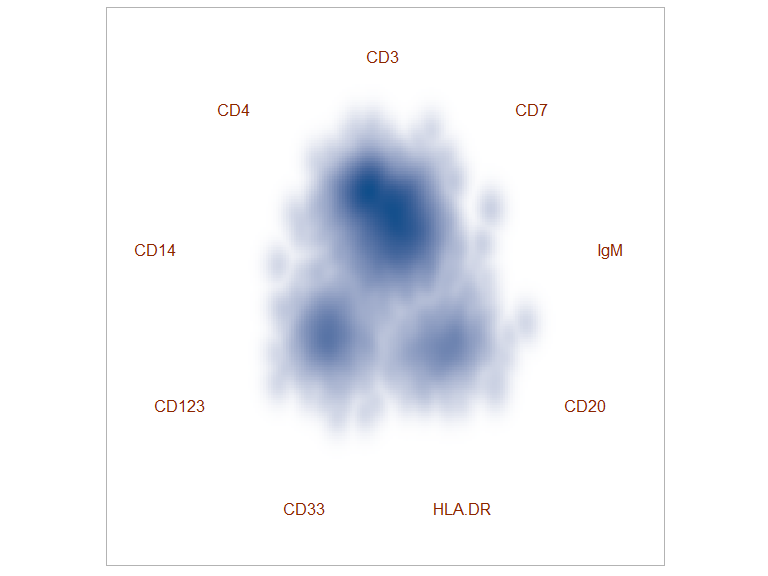smoothRadviz is actually a wrapper around stat_density2d, and returns the underlying ggplot object so that extra methods can be used:

smoothRadviz(ct.rv)+
geom_point(shape='.',alpha=1/5)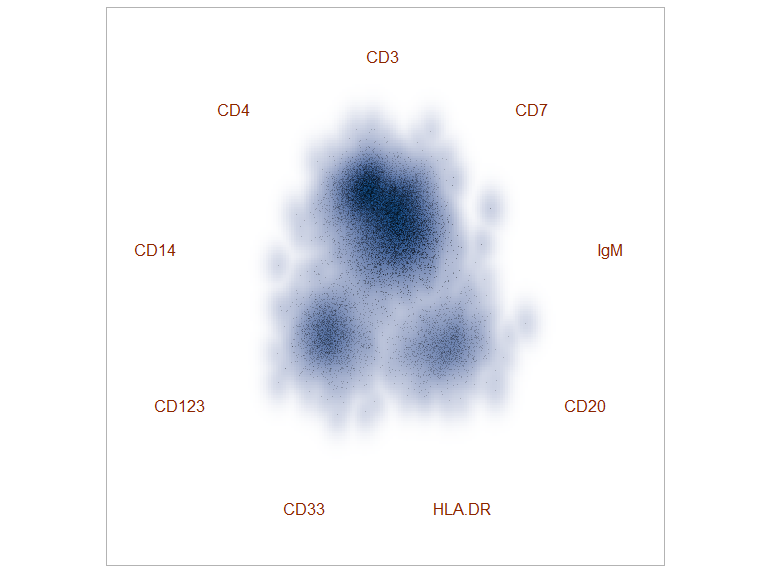Another alternative is contour plot, provided through the contour.radviz function:

contour(ct.rv)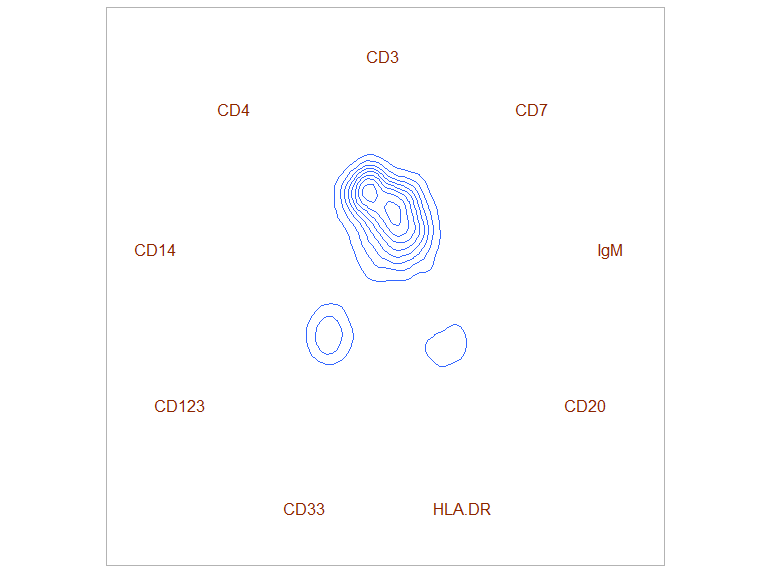This method can be used to highlight specific cell populations, in combination with subset.radviz:

cur.pop <- 'igm+'
sub.rv <- subset(ct.rv,refAnnots$Cells==cur.pop) smoothRadviz(ct.rv)+ geom_density2d(data=sub.rv$proj$data, aes(x=rx,y=ry), color='black')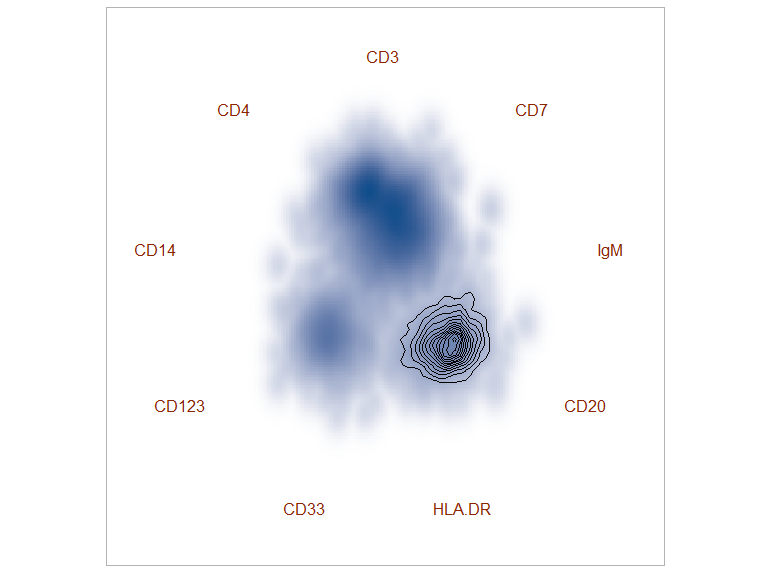The contour corresponds to igm+ cells, in the context of the full sample visualized as a smooth scatter. ## Visualizing Signal Intensity for Functional Channels Further insight can be gained from the use of hexagonal binning: hexplot(ct.rv)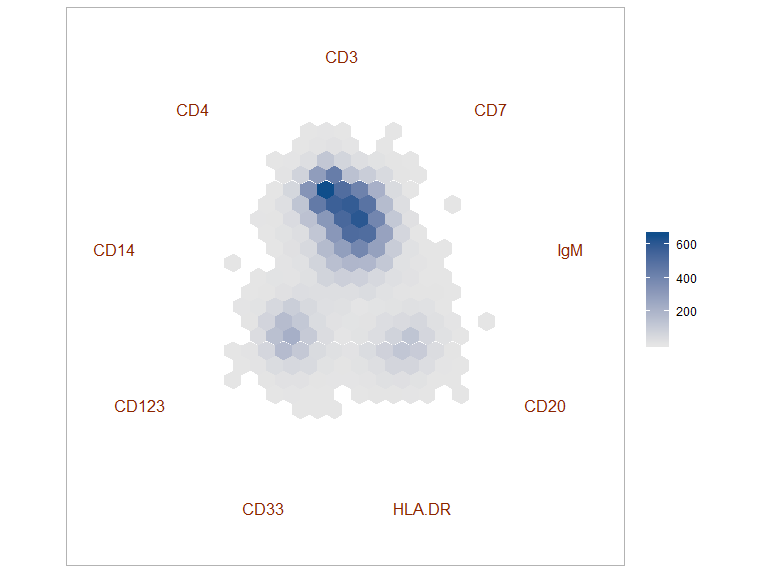Each bin can then be colored according to other dimensions in the dataset, such as CD4 signal intensity: hexplot(ct.rv,color='CD4')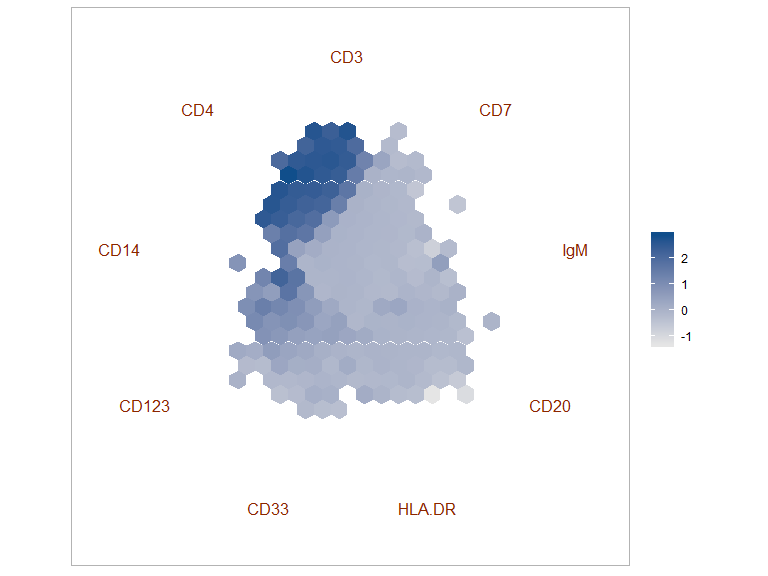But also S6 phosphorylation: hexplot(ct.rv,color='pS6')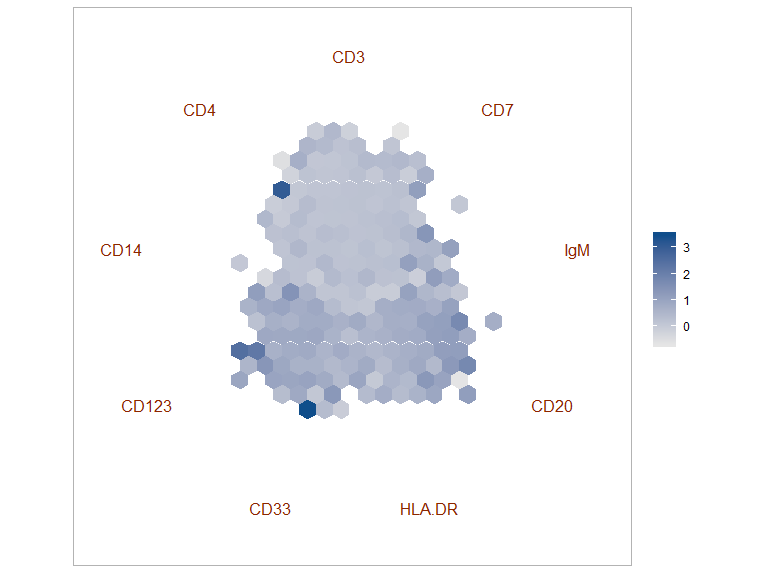To be compared with phospho-Akt signal: hexplot(ct.rv,color='pAkt')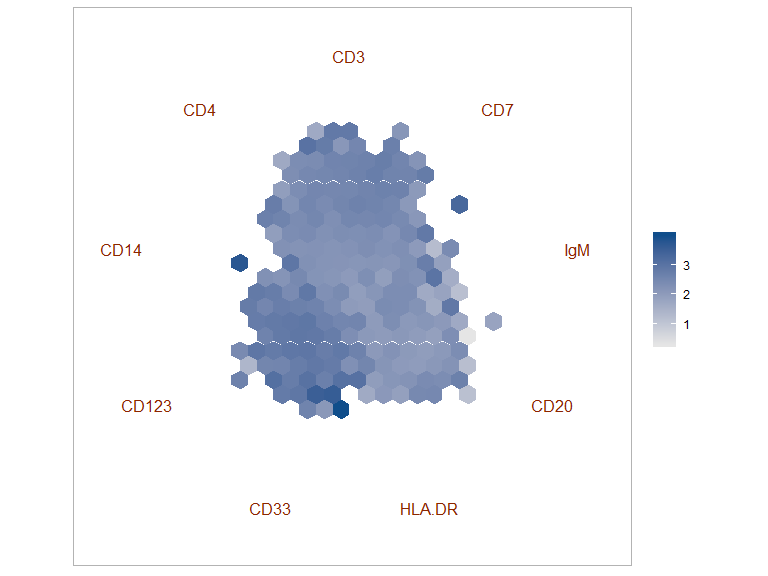And phospho-Erk signal: hexplot(ct.rv,color='pErk')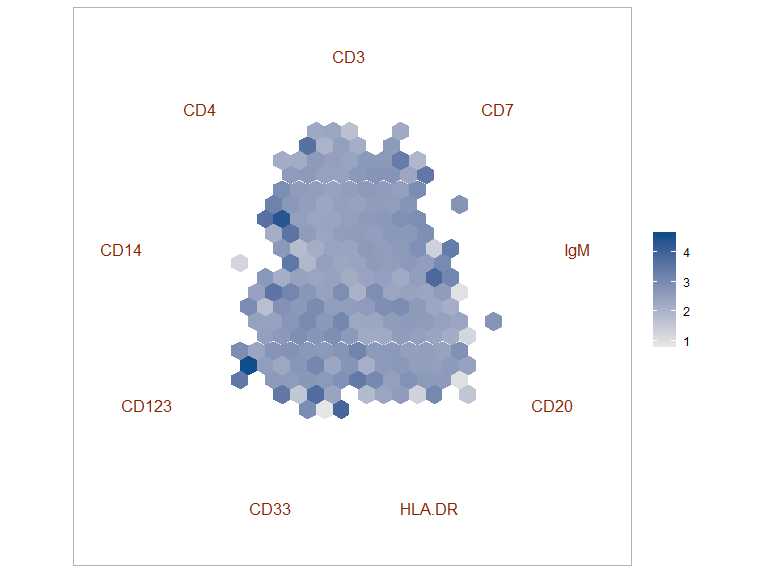## Visualizing Cell Populations Cells have been manually gated, leading to 14 sub-populations: ksink <- lapply(levels(refAnnots$Cells),function(x) cat(' *',x,'\n'))
• cd14-surf-
• cd14+surf-
• cd4+
• cd8+
• dendritic
• igm-
• igm+
• nk

To visualize the different populations, we compute a a bubble chart: the bubble position corresponds to the median signal intensity of each channel for this specific population, and the bubble size is proportional to the number of cells in this population:

bubbleRadviz(ct.rv,group = 'Cells')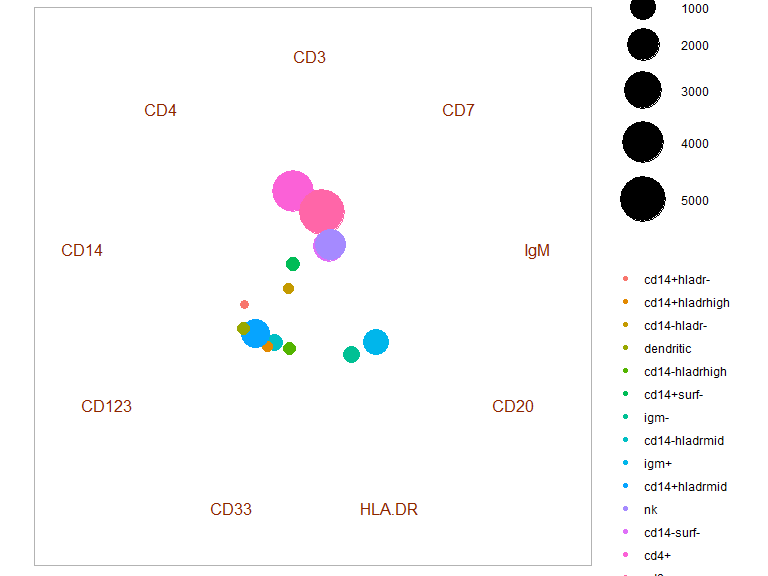Alternatively, bubbles can be colored after signal intensity of a functional channel, such as S6:

bubbleRadviz(ct.rv,group = 'Cells',color='pS6')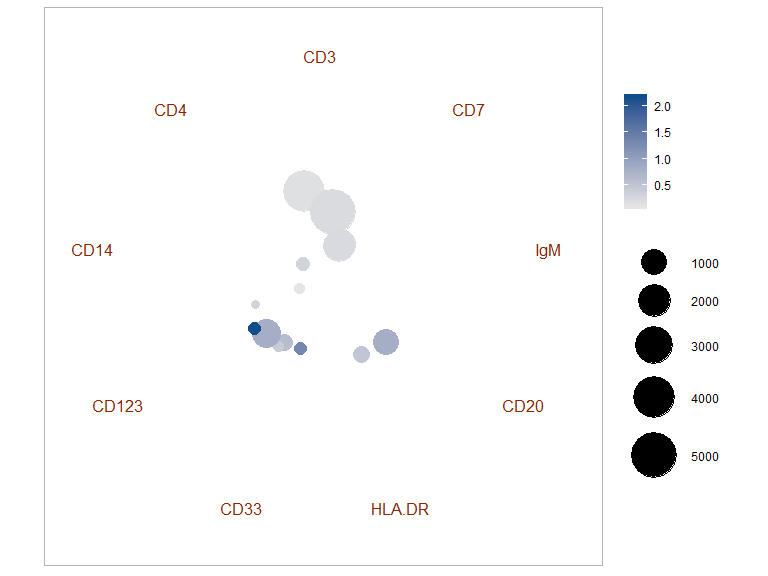## Visualizing Functional Changes

Radviz can also be used to visualize changes in functional space. We start by adding data from the Bodenmiller package.

data(untreatedPhenoMat)
data(untreatedFuncMat)
data(untreatedAnnots)
untreated.df <- bind_rows(ref.df %>%
mutate(Treatment='unstimulated',
Source=as.character(Source),
Cells=as.character(Cells)),
data.frame(untreatedAnnots,
untreatedPhenoMat,
untreatedFuncMat) %>%
mutate(Treatment=as.character(Treatment),
Source=as.character(Source),
Cells=as.character(Cells))) %>%
mutate(Treatment=factor(Treatment),
Treatment=relevel(Treatment,'unstimulated'),
Cells=factor(Cells))

For this analysis we will focus on CD4+ and CD8+ T cells:

tcells.df <- untreated.df %>%
filter(Cells %in% c('cd4+','cd8+'))
tcells.df %>%
count(Cells,Treatment)
## # A tibble: 8 x 3
##   Cells Treatment         n
##   <fct> <fct>         <int>
## 1 cd4+  unstimulated   3989
## 2 cd4+  BCR/FcR-XL     4076
## 3 cd4+  PMA/Ionomycin  4546
## 5 cd8+  unstimulated   5068
## 6 cd8+  BCR/FcR-XL     5098
## 7 cd8+  PMA/Ionomycin  5122
## 8 cd8+  vanadate       1200

Next we optimize the channel order, and create the projection:

func.S <- make.S(dimnames(refFuncMat)[])
func.sim <- cosine(as.matrix(tcells.df[,row.names(func.S)]))
optim.func <- do.optimRadviz(func.S,func.sim,iter=100,n=1000)
## Selected optimization function: in.da
## Starting performance: -55.9315
## 0 Current performance: -57.51255
## 1 Current performance: -57.70692
## 2 Current performance: -58.33536
## 3 Current performance: -58.53676
## 4 Current performance: -58.53676
## 5 Current performance: -58.67162
## 6 Current performance: -58.80389
## 7 Current performance: -58.80389
## 8 Current performance: -58.80389
## 9 Current performance: -58.80389
## 10 Current performance: -58.80389
## 11 Current performance: -58.80389
## Execution stopped after 11 iterations: no better solution found in the last 5 iterations
func.S <- make.S(tail(optim.func$best,1)[]) func.rv <- do.radviz(tcells.df,func.S,trans=trans) The overall T cell functional landscape is shown below: smoothRadviz(subset(func.rv,tcells.df$Treatment=='unstimulated'))+
facet_grid(~Cells)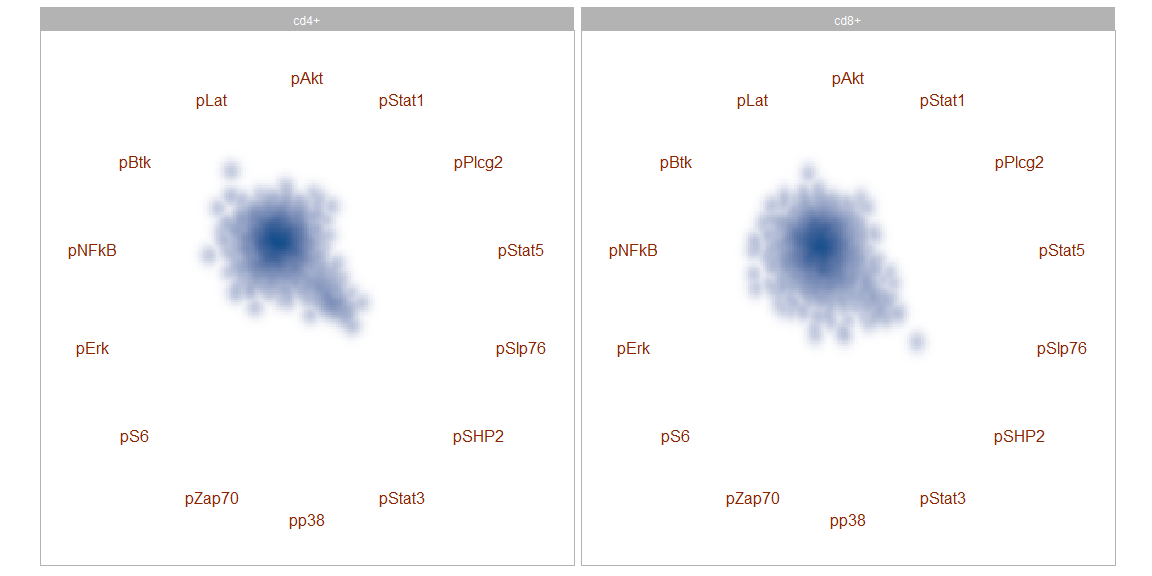The effect of different stimulations can then be visualized using contour plots:

plot(func.rv)+
geom_density2d(aes(color=Treatment))+
facet_grid(~Cells)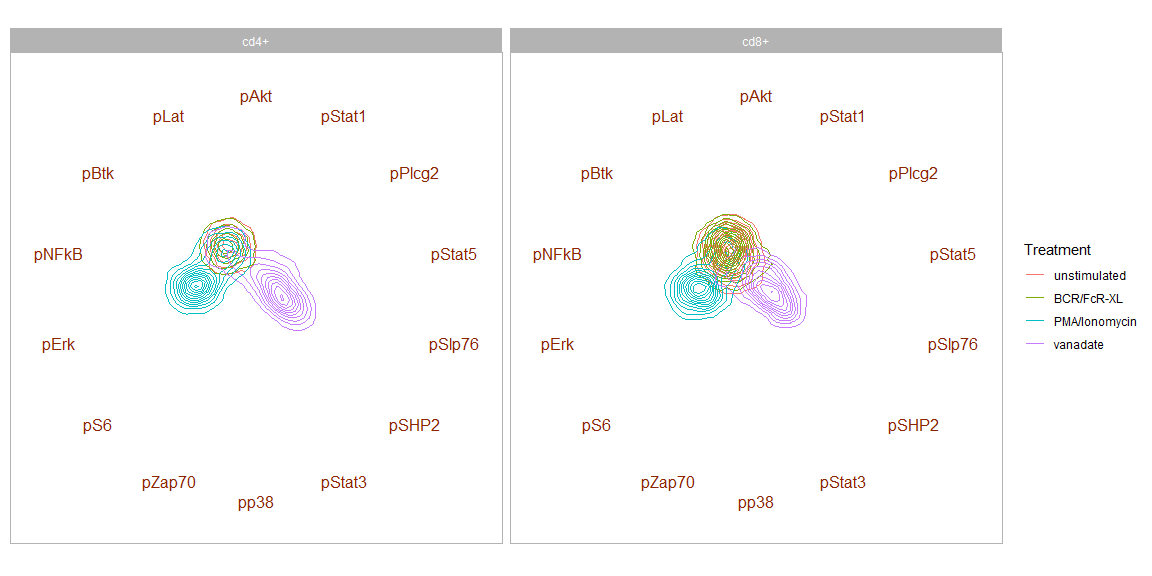Where one can see that BCR/FcR-XL has no effect and overlaps with unstimulated while PMA/Ionomycin increases pS6 and vanadate increases pSlp76:

tcells.df %>%
select(Cells, Treatment, pS6, pSlp76) %>%
gather('Channel','value',one_of('pS6','pSlp76')) %>%
ggplot(aes(x=Treatment,y=value))+
geom_boxplot(aes(fill=Treatment))+
facet_grid(Channel~Cells)+
theme_light()+
theme(axis.text.x=element_text(angle=45,hjust=1))